# Test: Analog & DE- 1

## 10 Questions MCQ Test GATE Electrical Engineering (EE) 2023 Mock Test Series | Test: Analog & DE- 1

Description
Attempt Test: Analog & DE- 1 | 10 questions in 30 minutes | Mock test for GATE preparation | Free important questions MCQ to study GATE Electrical Engineering (EE) 2023 Mock Test Series for GATE Exam | Download free PDF with solutions
QUESTION: 1

Solution:
QUESTION: 2

### Consider the following limiter circuit an input voltage V| = 10.5 sinlOOnt is applied. Assume that the diode drop is 0.7 V in forward bias. The zener breakdown voltage is 6.3 V.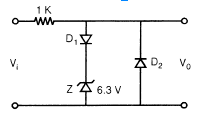The maximum and minimum values of the output voltage respectively are

Solution:

For +ve value of Vi, D1 is F.B and zener diode regulates the voltage at 6.3 V across it.
V0 = 6.3 + 0.7 = 7 V
and for negative half of V:, D2 is forward biased.
'    V0 = -0.7 V

QUESTION: 3

### In the saturation region of a BJT (Bipolar Junction Transistor)

Solution:

Both junctions could be forward biased.

QUESTION: 4

Given below is the Ic Vs VCE characteristic of two npn transistor P and Q having current gains β1 and β2 respectively.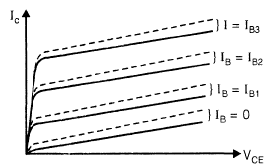Hard lines: Characteristics of P Dashed lines: Characteristics of Q

Which of the following is true?

Solution:

β of Q should be >> β of P.

QUESTION: 5

The thermal run-away in a CE-transistor amplifier can be prevented by biasing in such a wav that.

Solution:

It is derived from the basic condition.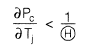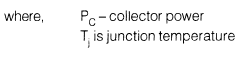QUESTION: 6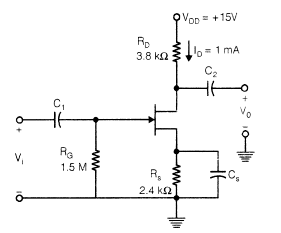IDSS = 8 mA Vp = -5 V, Assume Gate current » 0 all the capacitance used are of very large value. The voltage gain Av, is

Solution: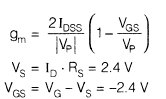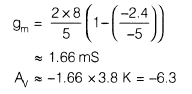QUESTION: 7

If the differential voltage gain and the common mode voltage gain of a differential amplifier are 50-dB and 2-dB resDectivelv. then its common mode rejection ratio is

Solution: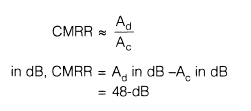QUESTION: 8

Which of the following instruments has a uniform scale?

Solution:

In the PMMC type instrument, the deflection is directly proportional to the current flowing through the instrument, we get a uniform scale for the instrument. It gives a uniform scale of upto 270° or more.

QUESTION: 9

Consider the following op-amp circuit with multiple stages: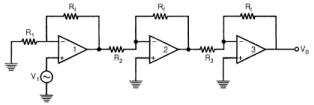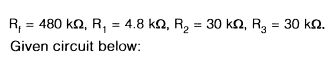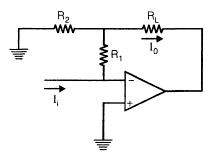Solution:

I0 = kIi (current controlled current source).

QUESTION: 10

Shown below is a positive feedback arrangement to generate sinusoidal oscillation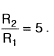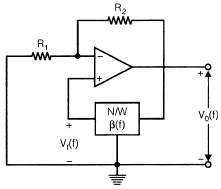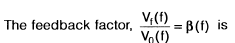Solution: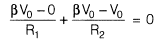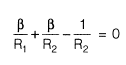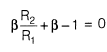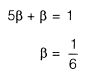Use Code STAYHOME200 and get INR 200 additional OFF Use Coupon Code